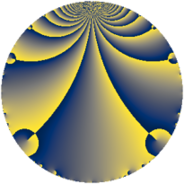Properties

 Label 6930.2.a.bwLevel $6930$ Weight $2$ Character orbit 6930.a Self dual yes Analytic conductor $55.336$ Analytic rank $1$ Dimension $2$ CM no Inner twists $1$

Related objects

Newspace parameters

 Level: $$N$$ $$=$$ $$6930 = 2 \cdot 3^{2} \cdot 5 \cdot 7 \cdot 11$$ Weight: $$k$$ $$=$$ $$2$$ Character orbit: $$[\chi]$$ $$=$$ 6930.a (trivial)

Newform invariants

 Self dual: yes Analytic conductor: $$55.3363286007$$ Analytic rank: $$1$$ Dimension: $$2$$ Coefficient field: $$\Q(\sqrt{3})$$ Defining polynomial: $$x^{2} - 3$$ Coefficient ring: $$\Z[a_1, \ldots, a_{13}]$$ Coefficient ring index: $$2$$ Twist minimal: no (minimal twist has level 2310) Fricke sign: $$1$$ Sato-Tate group: $\mathrm{SU}(2)$

$q$-expansion

Coefficients of the $$q$$-expansion are expressed in terms of $$\beta = 2\sqrt{3}$$. We also show the integral $$q$$-expansion of the trace form.

 $$f(q)$$ $$=$$ $$q + q^{2} + q^{4} - q^{5} - q^{7} + q^{8} +O(q^{10})$$ $$q + q^{2} + q^{4} - q^{5} - q^{7} + q^{8} - q^{10} - q^{11} + \beta q^{13} - q^{14} + q^{16} -\beta q^{17} - q^{20} - q^{22} + ( -2 - \beta ) q^{23} + q^{25} + \beta q^{26} - q^{28} + ( -2 + 2 \beta ) q^{29} + ( 4 - 2 \beta ) q^{31} + q^{32} -\beta q^{34} + q^{35} -\beta q^{37} - q^{40} -2 q^{41} - q^{44} + ( -2 - \beta ) q^{46} + 2 \beta q^{47} + q^{49} + q^{50} + \beta q^{52} -6 q^{53} + q^{55} - q^{56} + ( -2 + 2 \beta ) q^{58} -8 q^{59} + ( 2 + 2 \beta ) q^{61} + ( 4 - 2 \beta ) q^{62} + q^{64} -\beta q^{65} + ( -2 + \beta ) q^{67} -\beta q^{68} + q^{70} + ( -8 - 2 \beta ) q^{71} + ( 2 - 4 \beta ) q^{73} -\beta q^{74} + q^{77} -4 q^{79} - q^{80} -2 q^{82} + 4 \beta q^{83} + \beta q^{85} - q^{88} + ( -12 - \beta ) q^{89} -\beta q^{91} + ( -2 - \beta ) q^{92} + 2 \beta q^{94} -10 q^{97} + q^{98} +O(q^{100})$$ $$\operatorname{Tr}(f)(q)$$ $$=$$ $$2 q + 2 q^{2} + 2 q^{4} - 2 q^{5} - 2 q^{7} + 2 q^{8} + O(q^{10})$$ $$2 q + 2 q^{2} + 2 q^{4} - 2 q^{5} - 2 q^{7} + 2 q^{8} - 2 q^{10} - 2 q^{11} - 2 q^{14} + 2 q^{16} - 2 q^{20} - 2 q^{22} - 4 q^{23} + 2 q^{25} - 2 q^{28} - 4 q^{29} + 8 q^{31} + 2 q^{32} + 2 q^{35} - 2 q^{40} - 4 q^{41} - 2 q^{44} - 4 q^{46} + 2 q^{49} + 2 q^{50} - 12 q^{53} + 2 q^{55} - 2 q^{56} - 4 q^{58} - 16 q^{59} + 4 q^{61} + 8 q^{62} + 2 q^{64} - 4 q^{67} + 2 q^{70} - 16 q^{71} + 4 q^{73} + 2 q^{77} - 8 q^{79} - 2 q^{80} - 4 q^{82} - 2 q^{88} - 24 q^{89} - 4 q^{92} - 20 q^{97} + 2 q^{98} + O(q^{100})$$

Embeddings

For each embedding $$\iota_m$$ of the coefficient field, the values $$\iota_m(a_n)$$ are shown below.

For more information on an embedded modular form you can click on its label.

Label $$\iota_m(\nu)$$ $$a_{2}$$ $$a_{3}$$ $$a_{4}$$ $$a_{5}$$ $$a_{6}$$ $$a_{7}$$ $$a_{8}$$ $$a_{9}$$ $$a_{10}$$
1.1
 −1.73205 1.73205
1.00000 0 1.00000 −1.00000 0 −1.00000 1.00000 0 −1.00000
1.2 1.00000 0 1.00000 −1.00000 0 −1.00000 1.00000 0 −1.00000
 $$n$$: e.g. 2-40 or 990-1000 Significant digits: Format: Complex embeddings Normalized embeddings Satake parameters Satake angles

Atkin-Lehner signs

$$p$$ Sign
$$2$$ $$-1$$
$$3$$ $$-1$$
$$5$$ $$1$$
$$7$$ $$1$$
$$11$$ $$1$$

Inner twists

This newform does not admit any (nontrivial) inner twists.

Twists

By twisting character orbit
Char Parity Ord Mult Type Twist Min Dim
1.a even 1 1 trivial 6930.2.a.bw 2
3.b odd 2 1 2310.2.a.z 2

By twisted newform orbit
Twist Min Dim Char Parity Ord Mult Type
2310.2.a.z 2 3.b odd 2 1
6930.2.a.bw 2 1.a even 1 1 trivial

Hecke kernels

This newform subspace can be constructed as the intersection of the kernels of the following linear operators acting on $$S_{2}^{\mathrm{new}}(\Gamma_0(6930))$$:

 $$T_{13}^{2} - 12$$ $$T_{17}^{2} - 12$$ $$T_{19}$$ $$T_{23}^{2} + 4 T_{23} - 8$$ $$T_{29}^{2} + 4 T_{29} - 44$$ $$T_{31}^{2} - 8 T_{31} - 32$$

Hecke characteristic polynomials

$p$ $F_p(T)$
$2$ $$( -1 + T )^{2}$$
$3$ $$T^{2}$$
$5$ $$( 1 + T )^{2}$$
$7$ $$( 1 + T )^{2}$$
$11$ $$( 1 + T )^{2}$$
$13$ $$-12 + T^{2}$$
$17$ $$-12 + T^{2}$$
$19$ $$T^{2}$$
$23$ $$-8 + 4 T + T^{2}$$
$29$ $$-44 + 4 T + T^{2}$$
$31$ $$-32 - 8 T + T^{2}$$
$37$ $$-12 + T^{2}$$
$41$ $$( 2 + T )^{2}$$
$43$ $$T^{2}$$
$47$ $$-48 + T^{2}$$
$53$ $$( 6 + T )^{2}$$
$59$ $$( 8 + T )^{2}$$
$61$ $$-44 - 4 T + T^{2}$$
$67$ $$-8 + 4 T + T^{2}$$
$71$ $$16 + 16 T + T^{2}$$
$73$ $$-188 - 4 T + T^{2}$$
$79$ $$( 4 + T )^{2}$$
$83$ $$-192 + T^{2}$$
$89$ $$132 + 24 T + T^{2}$$
$97$ $$( 10 + T )^{2}$$